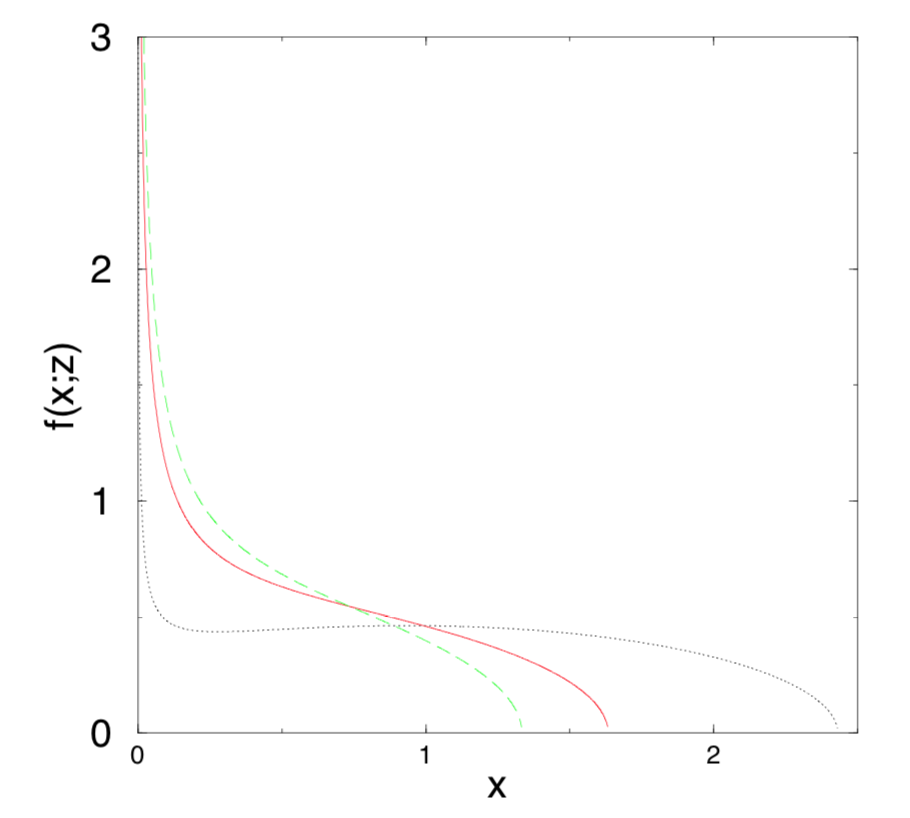# Eigenvalues of random matrix conditional on positive definiteness

Consider the Gaussian Orthogonal Ensemble, considered as a probability measure $$\mu$$ on the space of real symmetric matrices. Let $$\mu|PD$$ denote this measure conditioned on the event that the matrix is positive semi-definite. What if anything is known about this measure, and in particular about the distribution of the eigenvalues of a matrix drawn from this distribution?

The average density of states in the Gaussian ensembles whose eigenvalues are restricted to lie in a given interval, or to be greater than a given minimal value, was calculated by Dean and Majumdar in Extreme Value Statistics of Eigenvalues of Gaussian Random Matrices. The eigenvalue density has a $$1/\sqrt x$$ divergence at the end points of the interval. The case in the OP of a constraint of positive eigenvalues is the red solid curve in this plot from the cited paper.